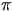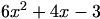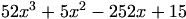Hard Maths Q Exam Type

Rocky

Posted 12 September 2005 - 04:08 PM

Q- A semi circular window of radius r cm is divided into 4 sectors. With each sector having an area of 125cm
(a) Show that the perimeter of each sector is
P= 2(r + 125/r)
(b) Find the minimum value of P

dfx

Posted 12 September 2005 - 05:19 PM

Right, I've attached a picture of what the semicircular window looks like. The question is rather scary at first site, but once you get to know the trick behind them, they're quite straightforward.Now, you're told that the Area of each sector is 125 cm.

Since they're equally divided in the semicircle (and hence the circle), you can assume that the total area of the circle divided by 8 (or the semicircular area divide by 4), will give you 125 cmThen the area of each sector, A = (r) / 8 (total circle area divide by 8.)

Substitute for values, and 125 = (r) / 8

Then, 1000 = (r) .

Just to digress slightly here. The trick to these is expressing the area in terms of something that you can then use for the perimeter. (i.e. something which is common to both area and perimeter.) Instinctively I'd jump to say radius, but look carefully at the final equation which you have to exress it as:

P= 2(r + 125/r)

Continued next mssg due to "too many emoticons error" which I think is rather annoying when you type out a huge answer. Mods?

dfx

Posted 12 September 2005 - 05:19 PM

Continued from above...

The value/symbol/variable that that final equation is lacking is... *drum roll*lol. SO, now back to where we left it, and you have to try to expressin terms of 1000 and r.

So we were at 1000 = (r)= 1000 / r.

Now concentrate on the perimeter. The perimeter of a circle is given by P = 2r, and in this case, the perimeter of the sector is given by:

P = (2r) / 8, and don't forget the 2 radii bounding the sides of the sector, so

P = ( 2r / 8 ) + 2rr / 4 + 2r.

So P =r / 4.

Now it's just a case of substituingwith the value that we ended up with from the area of the circle earlier, which was= 1000 / r.

So P = [ [ (1000 / r) r ] / 4 ] + 2r

= ( 1000r / 4r) + 2r

= ( 1000 / 4r ) + 2r.

= ( 250/r ) + 2r = 2r + 250/r

= 2(r + 125/r)

... Et Voila.

edit: for part 2 with the minimum value, I think you have to make r the subject for the formula and then proceed to differentiate with dr/dp, set it equal to zero, draw a nature table, and get your values. I'd wait for a confirmation.Dave

Posted 12 September 2005 - 05:37 PM

part b is easy enough we have done a few like this now

we are looking for which value of r will give the smallest value of P

the place to look is the fraction the best we can do is have 125/r = 1 which means r must = 125

means min value is 251

Ally

Posted 12 September 2005 - 05:53 PM

QUOTE(dfx @ Sep 12 2005, 06:19 PM)
Continued next mssg due to "too many emoticons error" which I think is rather annoying when you type out a huge answer. Mods?Just use the $$tags.dfx Posted 12 September 2005 - 05:57 PM [tex]?... I do use like [ p i ] and [ ^ 2 ] and all if that's what you mean... but I think they're interpreted as emoticons - well they are, technically.Ally Posted 12 September 2005 - 06:31 PM QUOTE(dfx @ Sep 12 2005, 06:57 PM) I do use like [ p i ] and [ ^ 2 ] and all if that's what you mean... but I think they're interpreted as emoticons - well they are, technically.Yeah they're emoticons. George/Steve installed tex notation which you can now use on the forum. It makes it easier for people to understand the maths with better communication.Just use the [tex] tags. i.e.====== CODE [tex]\pi$$

Gives you:Rocky

Posted 12 September 2005 - 07:09 PM

Substitute for values, and 125 = ( PIE r squared ) / 8

Then, 1000 = ( PIE r squared ) .

*Dfx how did you make 8 dissapear and then make the 125 into 1000?

dondon

Posted 12 September 2005 - 07:16 PM

I'm not dfx but

you want to transfer the 8 from one side to the other so you multiply 125 by 8 and you get 1000=rdfx

Posted 12 September 2005 - 07:34 PM

Spot on. Donna to the rescue.Ally, thanks for that, kept wondering how you did yours! lol. I'll keep it in mind.

The Wedge Effect

Posted 12 September 2005 - 07:48 PM

QUOTE(Ally @ Sep 12 2005, 07:31 PM)
QUOTE(dfx @ Sep 12 2005, 06:57 PM)
I do use like [ p i ] and [ ^ 2 ] and all if that's what you mean... but I think they're interpreted as emoticons - well they are, technically.Yeah they're emoticons.

George/Steve installed tex notation which you can now use on the forum. It makes it easier for people to understand the maths with better communication.Just use the $$tags. i.e.====== CODE [tex]\pi$$

Gives you:I didn't know that! That'd be useful!*tries out a few*Posted 15 September 2005 - 02:03 PM

Part b

The minimum value of P is found where 2(r + 125/r) is a minimum

ie P = 2r + 250r^-1

dP/dr = 2 - (250/r^2) = 0 at TP

(250/r^2) = 2

r^2 = 250/2 = 125

r = sqr(125)

Substitute this into P = 2(r + 125/r) will give 44.72cm.

Rocky

Posted 15 September 2005 - 04:24 PM

Thanx everyone for the help